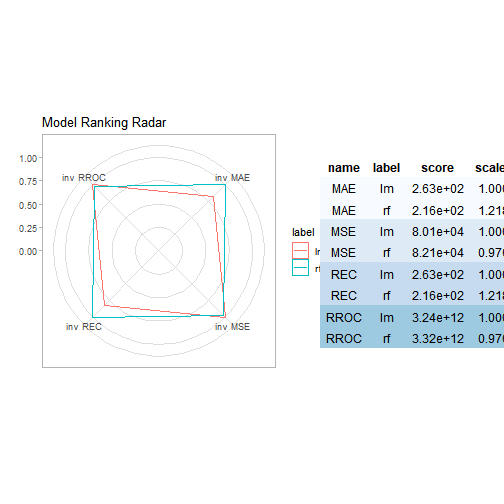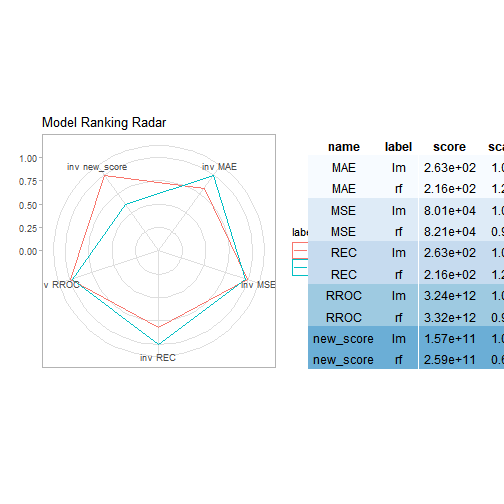# Regression use case - apartments data

To illustrate applications of auditor to regression problems we will use an artificial dataset apartments available in the DALEX package. Our goal is to predict the price per square meter of an apartment based on selected features such as construction year, surface, floor, number of rooms, district. It should be noted that four of these variables are continuous while the fifth one is a categorical one. Prices are given in Euro.

``````library(DALEX)
data("apartments")
``````
``````##   m2.price construction.year surface floor no.rooms    district
## 1     5897              1953      25     3        1 Srodmiescie
## 2     1818              1992     143     9        5     Bielany
## 3     3643              1937      56     1        2       Praga
## 4     3517              1995      93     7        3      Ochota
## 5     3013              1992     144     6        5     Mokotow
## 6     5795              1926      61     6        2 Srodmiescie
``````

# Models

## Linear model

``````lm_model <- lm(m2.price ~ construction.year + surface + floor + no.rooms + district, data = apartments)
``````

## Random forest

``````library("randomForest")
set.seed(59)
rf_model <- randomForest(m2.price ~ construction.year + surface + floor +  no.rooms + district, data = apartments)
``````

# Preparation for error analysis

The beginning of each analysis is creation of a `modelAudit` object. It’s an object that can be used to audit a model.

``````library("auditor")

lm_audit <- audit(lm_model, label = "lm", data = apartmentsTest, y = apartmentsTest\$m2.price)
rf_audit <- audit(rf_model, label = "rf", data = apartmentsTest, y = apartmentsTest\$m2.price)
``````

# Model Performance Audit

Model performance measures may be plotted together to easily compare model performances.

Function `modelPerformance()` compute chosen model performance measures. A result further from the center means a better model performance.

``````lm_mp <- modelPerformance(lm_audit, scores = c("MAE", "MSE", "REC", "RROC"))
rf_mp <- modelPerformance(rf_audit, scores = c("MAE", "MSE", "REC", "RROC"))

lm_mp
``````
``````##          score label name
## 1 2.633246e+02    lm  MAE
## 2 8.013798e+04    lm  MSE
## 3 2.632619e+02    lm  REC
## 4 3.244698e+12    lm RROC
``````

Results of `modelPerformance()` function for multiple models may be plotted together on one plot. Parameter `table` indicates whether the table with scores should be generated.

On the plot scores are inversed and scaled to [0,1].

``````plot(lm_mp, rf_mp, table = TRUE)
``````There is a possibiliy to define functions with custom model performance measure.

``````new_score <- function(object) sum((object\$residuals)^3)

lm_mp <- modelPerformance(lm_audit,
scores = c("MAE", "MSE", "REC", "RROC"),
new.score = new_score)

rf_mp <- modelPerformance(rf_audit,
scores = c("MAE", "MSE", "REC", "RROC"),
new.score = new_score)

plotModelRanking(lm_mp, rf_mp, table = TRUE)
``````# Other methods

Other methods and plots are described in vignettes: